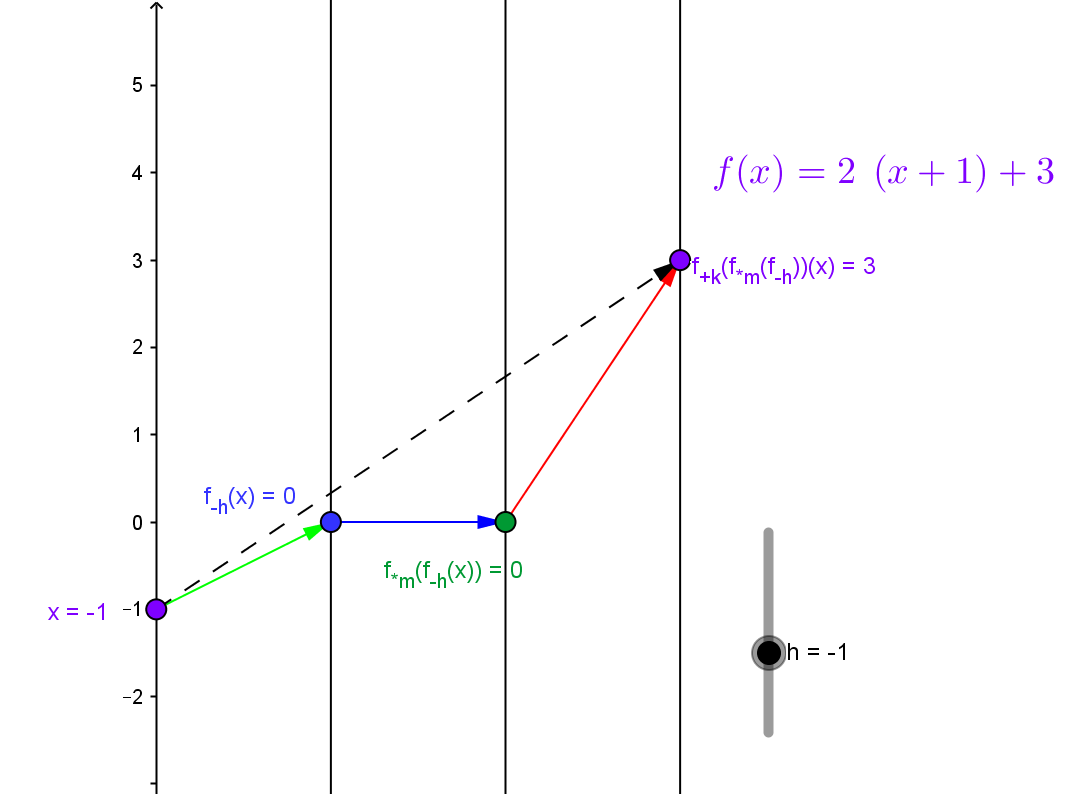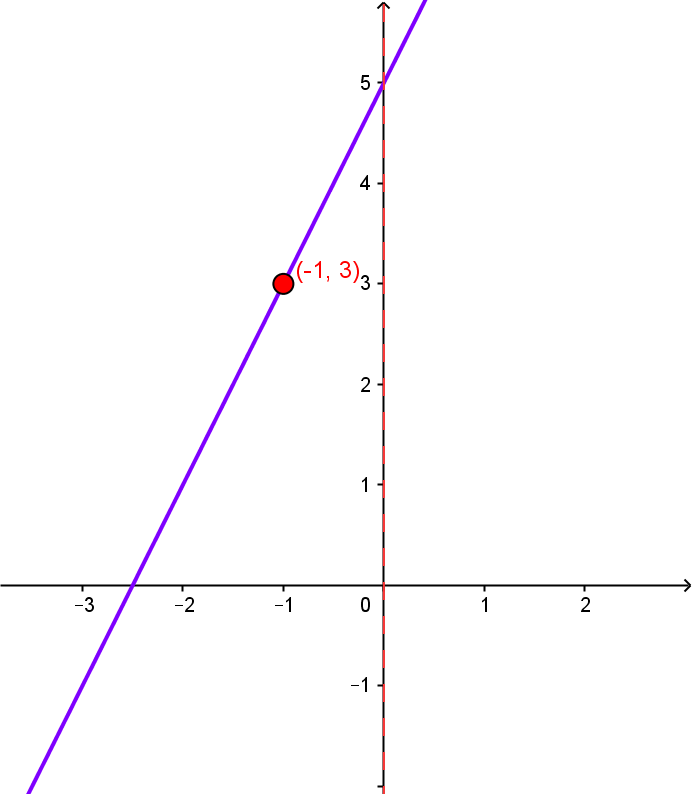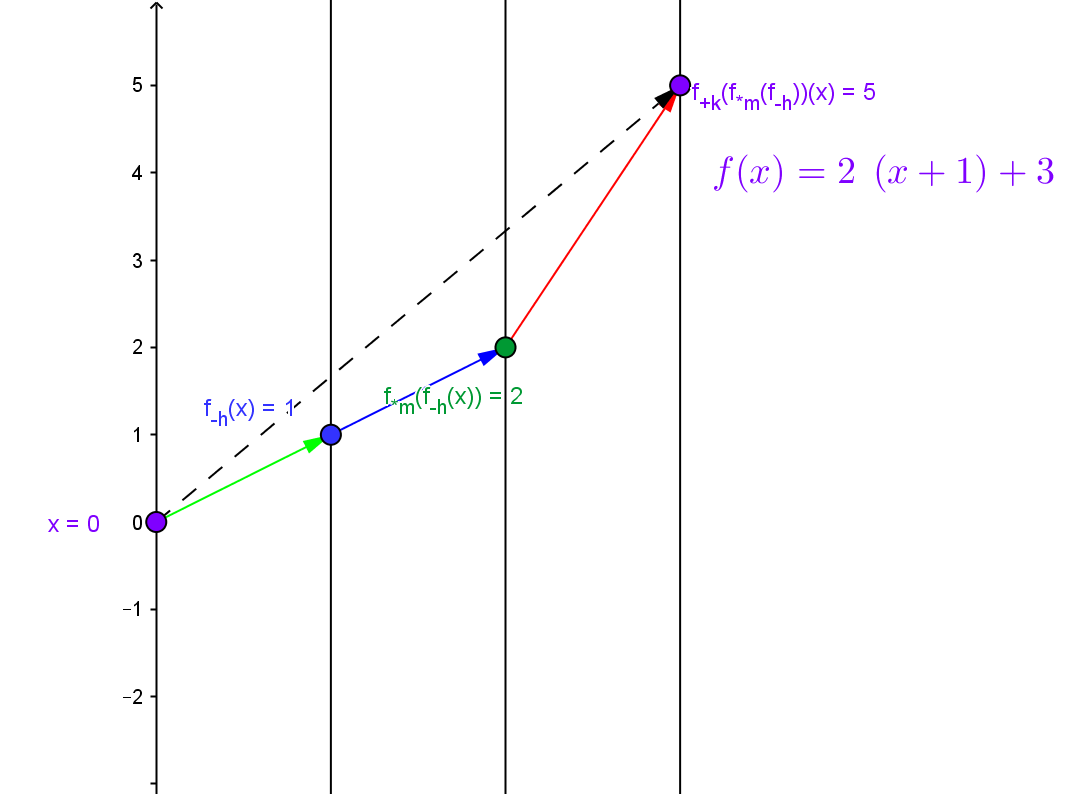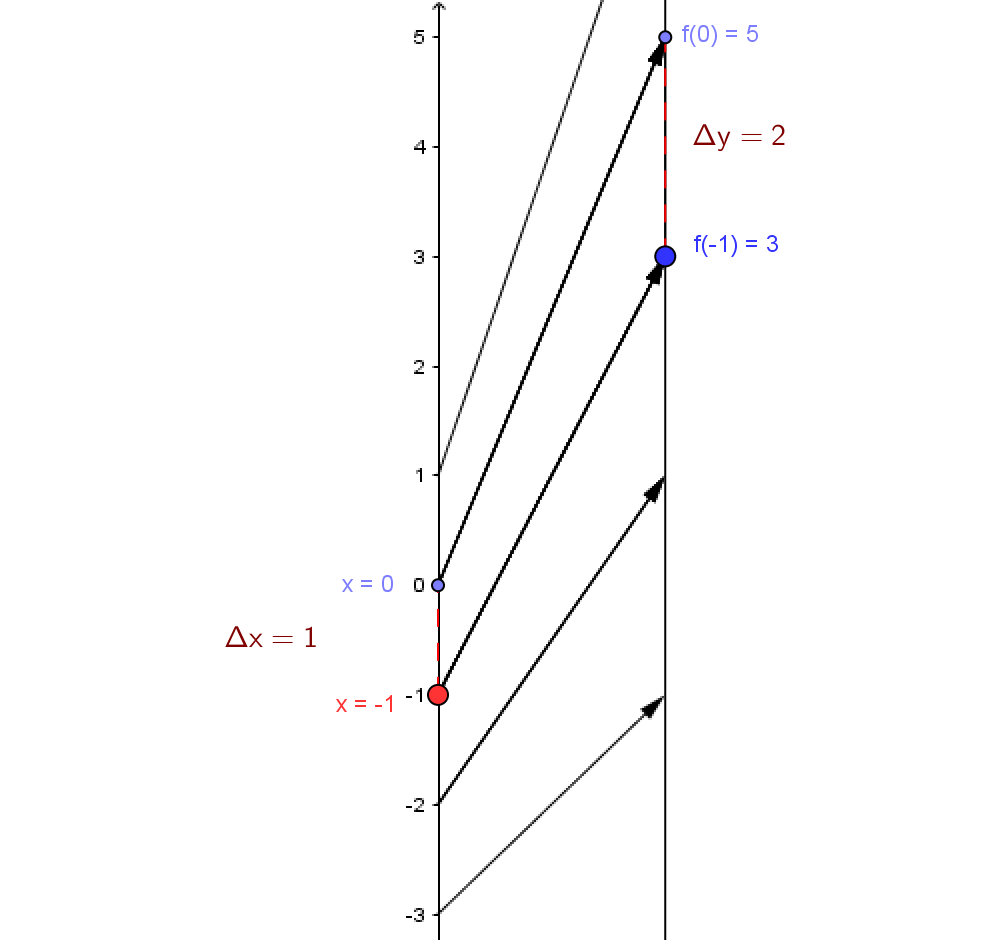Example LF.FORM.2: Suppose $f$ is a linear function with slope $=m=2$ and $f(−1)=3$. Find the point-slope form of the line. Visualize $f$ with a mapping diagram that illustrates the slope$= m=2$ and $f(−1)=3$. Find the X- and Y-intercepts for $f$ and visualize them on the mapping diagram as well.

Solution: Draw a mapping diagram yourself or use the GeoGebra figure.
Given a point / number, $x$, on the source line, there is a unique arrow  meeting the target line at the point / number, $3+2(x-(-1))= 2x+5$, which corresponds to the linear function's value for $x$

When the point in the domain is $-1$, the arrow points to $f(-1) = 3$ visualizing the point $(-1,3)$ on the graph of $f$.If we consider $f(0)= 2 +2(0+1) = 5$ then the difference in the value of $f$ for a unit change in $x$ is $\Delta y=f(0)-f(-1) = 5-3=2.$Since a unit step is used, we see the slope (magnification, rate) visualized in the gap between the heads of consecutive arrows on the mapping diagram.
As  $m > 0$, the arrows never cross illustrating that the function is increasing at a rate of 2 units on the target value for every unit increase in the domain value.

The X- intercept is the value $a$ on the domain axis from which the arrow hits the number $0$  on the target. For this function, that value is $a=-\frac 5 2$. You can check this by checking the box "Show a where f(a) = 0 " and entering $-\frac 5 2$ for $a$ on the GeoGebra figure.